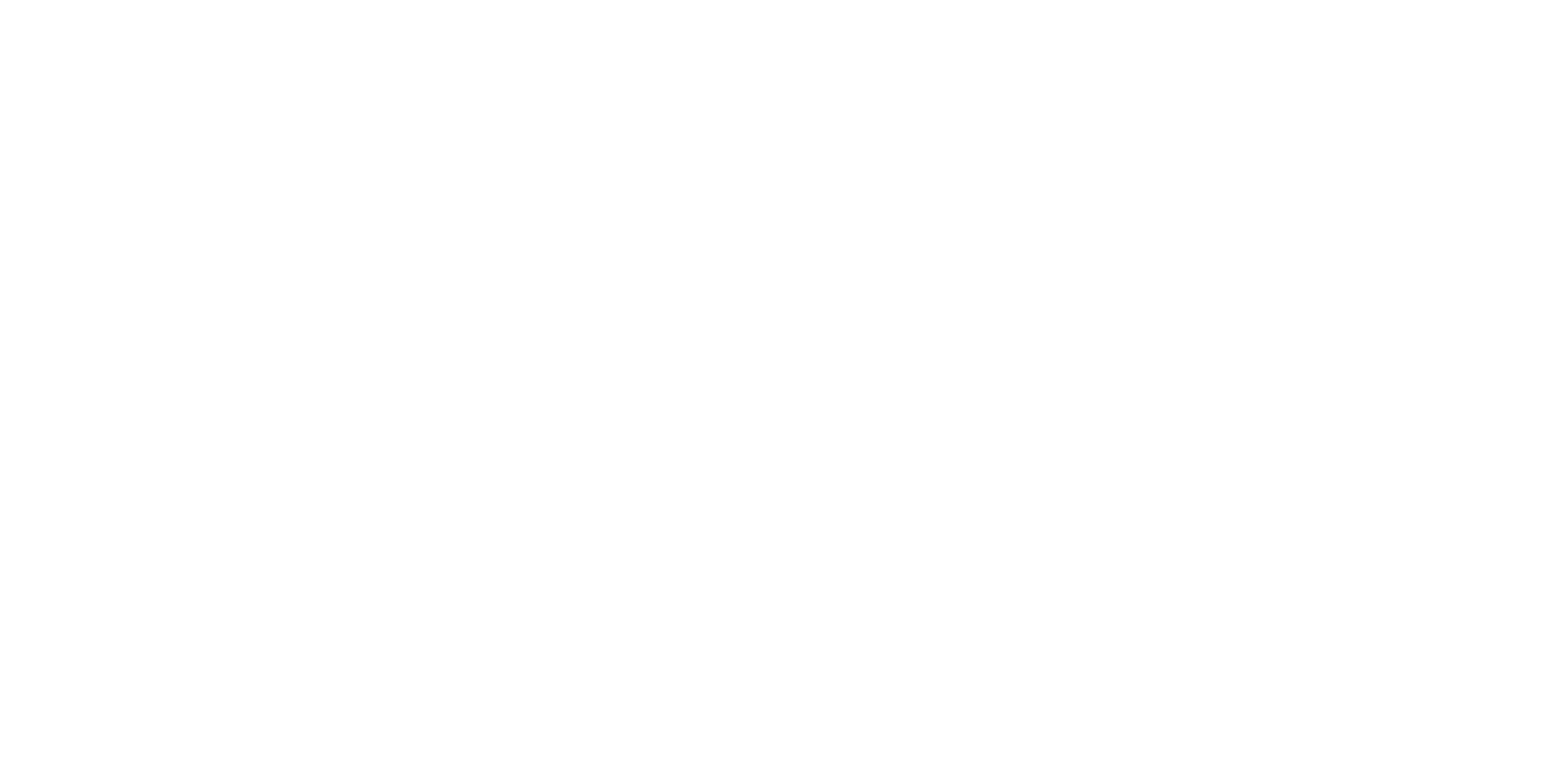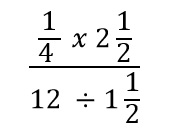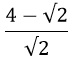Kuulchat

Online Education Resource Portal, its all freeWASSCE July 2018 mathematics

#### OBJECTIVE TEST

1.

Simplify:A.
5⁄8
B.
5⁄32
C.
5⁄64
D.
5⁄72

2.

Given that 3 x 91 + x=27-x,find the value of x.
A.
-35
B.
-123
C.
-2
D.
-3

3.

In a class of 39 student, 25 offer Fante and 19 offer Twi. Five student do not offer any of the two languages. How many student offer only Twi?
A.
6
B.
7
C.
8
D.
9

4.

Evaluate:A.
√2 - 1
B.
2√2 - 1
C.
√2 + 1
D.
2√2 + 1

5.

Mary and charity entered into a business partnership and ageed to share their porfit in the ratio 4 : 5 respectively. If Mary received Gh ¢ 5,000.00 less than Charity, how much profit did they make?
A.
GH¢30,000.00
B.
GH¢35,000.00
C.
GH¢40,000.00
D.
GH¢45,000.00

6.

A man bought a car which costs ₦5,000,000.00 from a dealer on hire purchase. He pays a deposit of ₦3,000,000.00 and agrees to pay the balance at 8% compound interest per annum. If he pays ₦1,000,000.00 at the end of each year, how much will be remaining to be paid after two years?
A.
₦92,800.00
B.
₦252,800.00
C.
₦320,000.00
D.
₦332,000.00

7.

y varies inversely as the square of x. When x = 3, y = 100. Find the value of x when y = 25.

A.

x = 30

B.

x = 12

C.

x = 6

D.

x = 5

8.

Solve the equation: t - 95 = -1115 .

A.

t = 3⁄5

B.

t = 11⁄15

C.

t = 4⁄5

D.

t = 13⁄15

9.

Find the truth set of the equation (x -2)2 + 3 = (x + 1)2 - 6.

A.

{-2}

B.

{-1}

C.

{1}

D.

{2}

10.

Two friends, Dede and Kofi decided, to buy the same type of car. They found out that the car cost ₦3,000,000.00. The amount of money (f) which Dede had was not enough to buy the car but Kofi had enough money (k) to buy the car. Which of the of the following inequalities is true?

A.

f≤₦3,000,000.00 ≤ k

B.

f> ₦3,000,000.00 > k

C.

f< ₦3,000,000.00 ≤ k

D.

f≥ ₦3,000,000.00 ≥ k

#### THEORY QUESTIONS

1.

(a)

Solve, correct to one decimal place, tan (θ + 25o) = 5.145, where 0o ≤ θ ≤ 90o.

(b)

In the relation t = m √(n2 + 4r):

(i)

make n the subject of the relation;

(ii)

find the positive value of n when t = 25, m = 5 and r = 4.

Show Solution
2.

The first three terms of an Arithmetic Progression (A.P:) are (x + 1),(4x - 2) and (6x - 3)

respectively.If the last term is 18, find the:

(a)

vale of x;

(b)

Sum of the terms of the progression.

Show Solution
3.

The angle of a sector of a circle with radius 22cm is 60o. If the sector is folded such that the straight edges coincide, forming a cone,calculate correct to one decimal place, the:

(a)